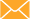info@sprayfoam.co.uk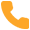0203 411 5188# Insulation Terminology Simplified

Understanding U-Values, R-Values and Thermal Conductivity to make informed home insulation decisions.### Thermal Conductivity

What is it?

When inquiring about home or commercial insulation, you may come across a term ‘thermal conductivity’, also known as, ‘Lambda’ these terms are the measure of how easily heat flows through types of material. Notably, the thermal conductivity of a specific type of material is separate from how thick the material is.

What does it prove?

Simply, the lower the thermal conductivity the better the thermal performance. A type of material with a low thermal conductivity means that heat will pass through it slower. Therefore, low thermal conductivity = better thermal performance.

How is it measured?

Thermal conductivity is measured using the abbreviation W/mK, which stands for, Watts per Metre Kelvin. Types of insulating materials have a thermal conductivity measurement between 0.008 W/mK (which is extremely good) to approximately 0.061 W/mK which is for basic insulating materials such as wood fibres.

Spray Foam Thermal Conductivity

For our Icynene spray foam insulation material, the thermal conductivity is 0.039 W/mK which shows a good level of thermal conductivity.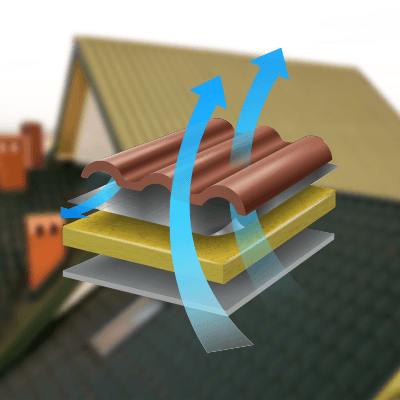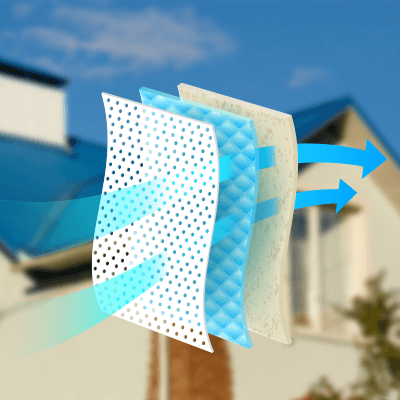### R-Value

What is it?

The R-Value, in laymen’s terms, can tell you how well any type of insulation can keep heat from leaving your home or property. Insulation R-Values are based on the following: type, thickness and density of the insulation material. The higher the R-Value of an insulator, the more thermal resistance it has and, therefore, the more superior insulating properties it holds.

How is it calculated?

The R-Value works in tandem with the thermal conductivity (see above) of an insulator. The formula for calculating the R-Value is:

R-Valuel
λ

l is the thickness of the insulating material (in metres) and λ is the thermal conductivity value in W/mK. For example, if you had 300mm (0.3m) of an insulator which had a thermal conductivity value of λ=1.1 the R-Value would be 0.3 / 1.1 = 0.27 m2K/W. The R-Value is a value that is measured in metres squared Kelvin per Watt (m2K/W).

Using the R-Value can be a great way to compare and contrast types of insulating and to calculate how beneficial adding types of insulation to your property can be. If you have the thermal conductivity of your insulating material, using the formula above, you can quickly assess the impact your insulation will have.

Spray Foam R-Value

Our open-cell foam insulation product has an R-Value of 3.7 per inch, whereas our closed-cell foam insulation has an R-Value of 7 per inch (you can learn more about our open cell and closed cell insulation here). Our professionals recommend at least 2 inches of closed-cell foam per application which equates to an R-Value of at least 14.

### U-Value

What is it?

The U-Value is similar to the R-Value but a more in-depth and accurate assessment. It measures how much heat is lost through a material, but unlike the R-Value, it includes three practices in which heat is lost: conduction, convection and radiation. The R-Value only considers one of the three, the conduction. For instance, the U-Value takes into account environmental temperatures, if a building is being heated and the building is located in a cold climate, heat will be flowing via radiation and convection, the U-Value uses these additional thermal resistances and analyses interior and exterior surfaces of each element. These resistances are referred to as Rsi and Rso respectively. The main concept to remember with the U-Value is, the lower the value, the better the material acts as a heat insulator.

How is the U-Value calculated?

We can calculate the U-Value by using the reciprocal of the R-Value and then by adding the convection and radiation heat losses.

U-Value=1+(Convection Heat Losses + Radiation Heat losses)
R-Value

The U-Value is measured in Watts per metre squared Kelvin W/m2K. If we were to have a frame of reference, or a guideline for a U-Value, we can look at the typical uninsulated cavity wall, which has an estimated U-Value of 1.6W/m2K or an uninsulated solid wall, which has a U-Value of 2 W/m2K. Due to the precise and complicated nature of the U-Value, our professionals always recommend using a U-Value software to work out your analysis.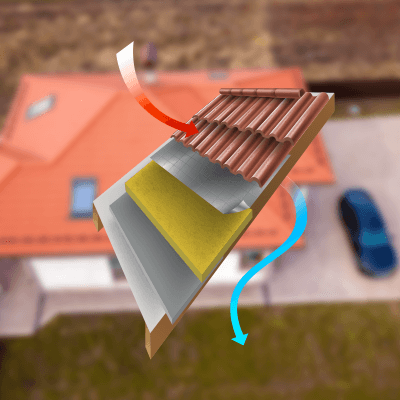#### Analysis Summary

To conclude, we have discussed three key terms regarding property insulation: thermal conductivity, R-Values and U-Values. Some key points to remember and to refer to next time you are inquiring about home or commercial insulation would be that:

1. The lower the value of thermal conductivity, the better.
2. The higher the R-Value, the more thermal resistance the material has.
3. The lower the U-Value, the better the material acts as a heat insulator.
4. The U-Value provides the most accurate assessment of a material’s insulation.